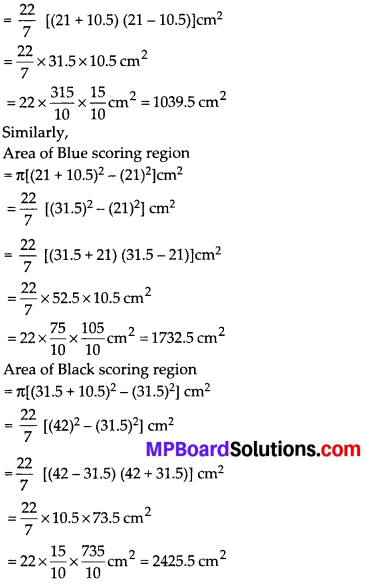# MP Board Class 10th Maths Solutions Chapter 12 Areas Related to Circles Ex 12.1

In this article, we will share MP Board Class 10th Maths Book Solutions Chapter 12 Areas Related to Circles Ex 12.1 Pdf, These solutions are solved subject experts from the latest edition books.

## MP Board Class 10th Maths Solutions Chapter 12 Areas Related to Circles Ex 12.1

Unless stated otherwise, use π = $$\frac{22}{7}$$.

Question 1.
The radii of two circles are 19 cm and 9 cm respectively. Find the radius of the circle which has circumference equal to the sum of the circumferences of the two circles.
Solution:
We have, r1 = 19 cm and r2 = 9 cm
∴ Circumference of circle – I = 2πr1 = 2π(19) cm
and circumference of circle – II = 2πr2 = 2π(9) cm
Sum of the circumferences of circle-I and circle-II
= 2π(19) cm + 2π(9) cm
= 2π(19 + 9) cm
= 2π(28) cm
Let R be the radius of the circle – III.
∴ Circumference of circle – III = 2πR
According to the condition, 2πR = 2π(28)
⇒ R = $$\frac{2 \pi(28)}{2 \pi}$$ = 28 cm
Thus, the radius of the new circle = 28 cm.Question 2.
The radii of two circles are 8 cm and 6 cm respectively. Find the radius of the circle having area equal to the sum of the areas of the two circles.
Solution:
We have,
Radius of circle – I, r1 = 8 cm
Radius of circle – II, r2 = 6 cm
∴ Area of circle – I = πr12 = π(8)2 cm2
Area of circle-II = πr22 = π(6)2 cm2
Let the radius of the circle – III be R cm.
∴ Area of circle-III = πR2
Now, according to the condition,
πr12 + πr22 = πR2
⇒ π(8)2 + π(6)2 = πR2
⇒ π(82 + 62) = πR2
⇒ 82 + 62 = R2
⇒ 64 + 36 = R2
⇒ 100 = R2
⇒ 102 = R2 ⇒R = 10
Thus, the radius of the new circle = 10 cm.

Question 3.
The given figure depicts an archery target marked with its five scoring regions from the centre outwards as Gold, Red, Blue, Black and White. The diameter of the region representing Gold score is 21 cm and each of the other bands is 10.5 cm wide. Find the area of each of the five scoring regions.Solution:
Diameter of the innermost (Gold scoring) region = 21 cm
Radius of the innermost (Gold scoring) region = $$\frac{21}{2}$$ = 10.5 cm
∴ Area of Gold scoring region = π(10.5)2 cm2
= $$\frac{22}{7} \times\left(\frac{105}{10}\right)^{2} \mathrm{cm}^{2}=\frac{22}{7} \times \frac{105}{10} \times \frac{105}{10} \mathrm{cm}^{2}$$
= $$\frac{22 \times 15 \times 105}{100}$$ cm2 = 346.50 cm2
Since, each band is 10.5 cm wide.
∴ Radius of Red scoring region
= 10.5 cm + 10.5 cm
= 21 cm
Area of Red scoring region
= π(10.5 + 10.5)2 cm2 – π(10.5)2 cm2
= [π(21)2 – π(10.5)2] cm2
= π[(21)2 – (10.5)2] cm2Area of White scoring region
= π[(42 + 10.5)2 – (42)2] cm2
= π[(52.5)2 – (42)2] cm2
= π[(52.5 + 42)(52.5 – 42)] cm2
= $$\frac{22}{7}$$ × 94.5 × 10.5 = 22 × $$\frac{945}{10} \times \frac{15}{10}$$
= 3118.5 cm2

Question 4.
The wheels of a car are of diameter 80 cm each. How many complete revolutions does each wheel make in 10 minutes when the car is travelling at a speed of 66 km per hour?
Solution:
Diameter of a wheel = 80 cm
∴ Radius of the wheel = $$\frac{80}{2}$$ cm = 40 cm
So, circumference of the wheel
= 2πr = 2 × $$\frac{22}{7}$$ × 40 cm
⇒ Distance covered by a wheel in one revolution = $$\frac{2 \times 22 \times 40}{7}$$ cm
Distance travelled by the car in 1 hour (i.e., in 60 mins)
= 66 km = 66 × 1000 × 100 cm
∴ Distance travelled in 10 minutesThus, the required number of revolutions = 4375Question 5.
Tick the correct answer in the following and justify your choice: If the perimeter and the area of a circle are numerically equal, then the radius of the circle is
(A) 2 units
(B) π units
(C) 4 units
(D) 7 units
Solution:
(A): We have
Numerical area of the circle
= Numerical circumference of the circle
⇒ πr2 = 2πr
⇒ πr2 – 2πr = 0
⇒ r2 – 2r = 0
⇒ r(r – 2) = 0
⇒ r = 0 or   r = 2
But r cannot be zero
∴ r = 2
Thus, the radius of circle is 2 units.